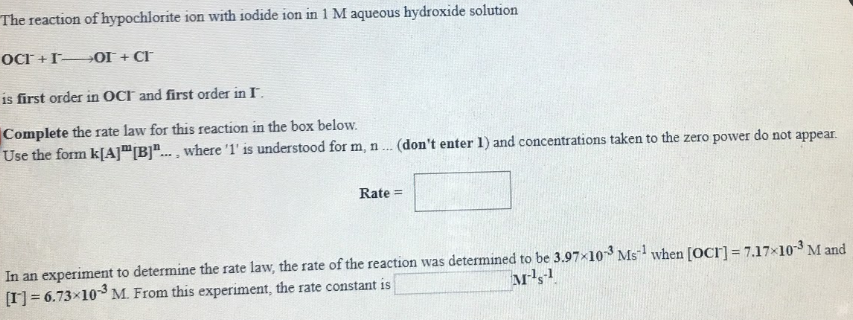# The reaction of hypochlorite ion with iodide ion in 1 M aqueous hydroxide solution OCl^- + I^- → OI^- + Cl^- is first order in OCl^- and first order in I^- Complete the rate law for this reaction in the box below. Use the form k[A]^m[B]^n... where '1' is understood for m, n... (don't enter I) and concentrations taken to the zero power do not appear. In an experiment to determine the rate law, the rate of the reaction was determined to be 3.97x10^-3 Ms^-1 when [OCl^-] = 7.17x10^-3 M and [I^-] = 6.73x10^-3 M. From this experiment, the rate constant is ____ M^-1s^-1